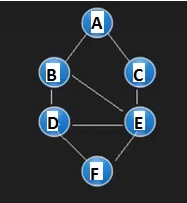# Generate a graph using Dictionary in Python

PythonProgrammingServer Side Programming

The graphs can be implemented using Dictionary in Python. In the dictionary, each key will be the vertices, and as value, it holds a list of connected vertices. So the entire structure will look like Adjacency list of a graph G(V, E).

We can use the basic dictionary object, but we are using default dict. It has some additional features. It has one additional writable instance variable.

We are providing a text file, which contains the number of vertices, number of edges, names of vertices, and the list of edges. For undirected graph, we are providing two edges like (u,v) and (v,u).

We are using this graph in this example.The file for the graph is like below −

Graph_Input.txt

6
8
A|B|C|D|E|F
A,B
B,A
A,C
C,A
B,D
D,B
B,E
E,B
C,E
E,C
D,E
E,D
D,F
F,D
E,F
F,E


So at first, we are taking the names of the vertices, and then read the edges to insert into list.

## Example code

from collections import defaultdict
defcreate_graph(filename):
graph = defaultdict(list) #create dict with keys and corresponding lists
with open(filename, 'r') as graph_file:
vert_Names = vert_Names.rstrip('\n') #Remove the trailing new line character
nodes = vert_Names.split('|') #Cut the vertex names
for node in nodes: #For each vertex, create empty list
graph[node] = []
#Read edges from file and fill the lists
for line in graph_file:
line = line.rstrip('\n') #Remove the trailing new line character
edge = line.split(',')
graph[edge].append(edge) #The edge is source and edge is dest
return graph
my_graph = create_graph('Graph_Input.txt')
for node in my_graph.keys(): #Print the graph
print(node + ': ' + str(my_graph[node]))


## Output

A: ['B', 'C']
B: ['A', 'D', 'E']
C: ['A', 'E']
D: ['B', 'E', 'F']
E: ['B', 'C', 'D', 'F']
F: ['D', 'E']


Now we will see some basic operations on the given graph G(V,E). At first we will see how to get a path from source vertex to destination vertex. The given code is a part of this operation. To execute it, you have to generate graph using the previous method.

## Example code

#Function to find path from source to destination
defget_path(graph, src, dest, path = []):
path = path + [src]
if src == dest: #when destination is found, stop the process
return path
for vertex in graph[src]:
if vertex not in path:
path_new = get_path(graph, vertex, dest, path)
if path_new:
return path_new
return None
my_graph = create_graph('Graph_Input.txt')
path = get_path(my_graph, 'A', 'C')
print('Path From Node A to C: ' + str(path))


## Output

Path From Node A to C: ['A', 'B', 'D', 'E', 'C']


Now we will see how to get all possible paths from Source Vertex to Destination Vertex. The given code is a part of this operation. To execute it, you have to generate graph using the previous method.

## Example code

#Function to find all paths from source to destination
defget_all_path(graph, src, dest, path = []):
path = path + [src]
if src == dest: #when destination is found, stop the process
return [path]
paths = []
new_path_list = []
for vertex in graph[src]:
if vertex not in path:
new_path_list = get_all_path(graph, vertex, dest, path)
for new_path in new_path_list:
paths.append(new_path)
return paths
my_graph = create_graph('Graph_Input.txt')
paths = get_all_path(my_graph, 'A', 'C')
print('All Paths From Node A to C: ')
for path in paths:
print(path)


## Output

All Paths From Node A to C:
['A', 'B', 'D', 'E', 'C']
['A', 'B', 'D', 'E', 'C']
['A', 'B', 'D', 'F', 'E', 'C']
['A', 'B', 'D', 'F', 'E', 'C']
['A', 'B', 'D', 'F', 'E', 'C']
['A', 'B', 'E', 'C']
['A', 'C']


Finally, we will see how to get the shortest path from source to destination vertex. The given code is a part of this operation. To execute it, you have to generate graph using the previous method.

## Example code

#Function to find shortest path from source to destination
def get_shortest_path(graph, src, dest, path = []):
path = path + [src]
if src == dest: #when destination is found, stop the process
return path
short = None
for vertex in graph[src]:
if vertex not in path:
new_path_list = get_shortest_path(graph, vertex, dest, path)
if new_path_list:
if not short or len(new_path_list) <len(short):
short = new_path_list
return short
my_graph = create_graph('Graph_Input.txt')
path = get_shortest_path(my_graph, 'A', 'C')
print('Shortest Paths From Node A to C: ' + str(path))


## Output

Shortest Paths From Node A to C: ['A', 'C']